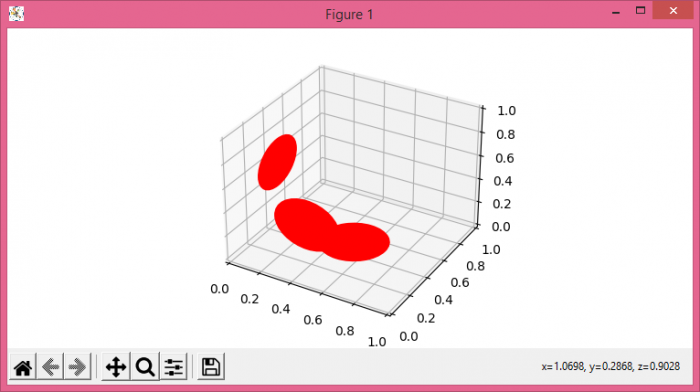# How to plot a 3D patch collection in matplotlib?

To plot a 3D patch collection in matplotlib, we can take the following steps −

• Set the figure size and adjust the padding between and around the subplots.
• Create a new figure or activate an existing figure.
• Get the current axes and set projection as 3d.
• Iterate ["x", "y", "z"] list, and set the circle patch using pathpatch_2d_to_3d() method to convert a PathPatch to a PathPatch3D object.
• To display the figure, use show() method.

## Example

import matplotlib.pyplot as plt
from matplotlib.patches import Circle
import mpl_toolkits.mplot3d.art3d as art3d

plt.rcParams["figure.figsize"] = [7.50, 3.50]
plt.rcParams["figure.autolayout"] = True

fig = plt.figure()
ax = fig.gca(projection='3d')

for i in ["x", "y", "z"]:
cir = Circle((0.5, 0.5), 0.2, color='red')
plt.show()